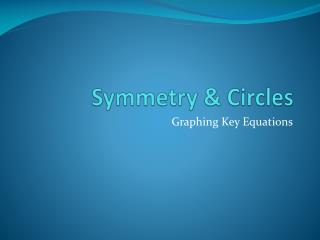# Symmetry & Circles - PowerPoint PPT PresentationDownload PresentationSymmetry & Circles

Symmetry & CirclesDownload Presentation## Symmetry & Circles

- - - - - - - - - - - - - - - - - - - - - - - - - - - E N D - - - - - - - - - - - - - - - - - - - - - - - - - - -
##### Presentation Transcript

1. Symmetry & Circles Graphing Key Equations

2. What is Symmetry? Symmetry

3. Symmetry with Respect to x-Axis If the graph contains the point (x, y), then must contain the point (x, -y). Remember that you are folding it over a horizontal line therefore the y value is changing (folding down).

4. Symmetry with Respect to y-axis If the graph contains the point (x, y), then it must also contain the point (-x, y). Remember you are folding it over a vertical line, therefore the x is going to change (folding one side over the other).

5. Symmetry with Respect to the Origin If the graph contains the point (x, y), then it must also contain the point (-x, -y). Remember, you are folding the graph both horizontally and vertically. This changes both the x and y values.

6. Testing for Symmetry X-Axis: Replace y by –y in equation. (Will only be true if there is a y^2 somewhere in the equation. Y-Axis: Replace x by –x in the equation. Origin: Replace x by –x and y by –y.

7. Circles Definition of Circle

8. Circles Earth is represented on a map of the solar system so that its surface is the circle with equation x^2+y^2 +2x +4y -4091 = 0. What does this all mean?

9. Equation of a Circle How to find the equations of a circle.

10. Circles Write an equation for the path of a communications satellite in a circular orbit 22,000 miles above the earth. (Assume that the radius of the earth is 4000 miles.) How would this equation change if the orbit is 30,000 miles above the earth?